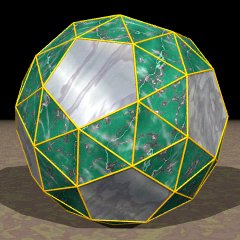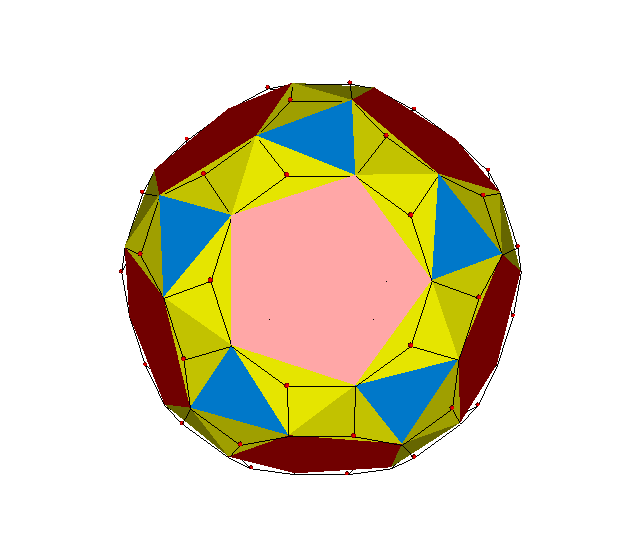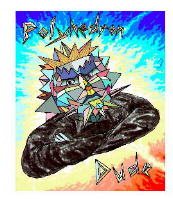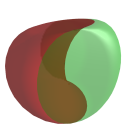Acronym snid TOCID symbol sID Name snub dodecahedron,snub icosidodecahedron `© ©` Circumradius sqrt[162+42 sqrt(5)+6 cbrt[20448+9140 sqrt(5)+12 sqrt(7137+3192 sqrt(5))]+6 cbrt[20448+9140 sqrt(5)-12 sqrt(7137+3192 sqrt(5))]]/12 = 2.155837 Vertex figure [34,5] Snub derivation `` General of army (is itself convex) Colonel of regiment (is itself locally convex – no other uniform polyhedral members) Dihedral angles between {3} and {3}:   arccos([cbrt(u+v)+cbrt(u-v)+1/3]/3) = 164.175366° between {3} and {5}:   ≈152.930° where u = -2 (49+27 sqrt(5))/27 v = 2 sqrt[2 (93+49 sqrt(5))/3]/3 Confer compounds: dissid ExternallinksAs abstract polytope snid is isomorphic to gosid, gisid, and girsid, thereby replacing prograde icosahedral triangles and pentagons respectively by prograde icosahedral triangles and (prograde) pentagrams, by prograde icosahedral triangles and retrograde pentagrams, by retrrograde icosahedral triangles and retrograde pentagrams.

Incidence matrix according to Dynkin symbol

```s3s5s

demi( . . . ) | 60 |  1  2  2 |  1  1  3
--------------+----+----------+---------
s 2 s   ♦  2 | 30  *  * |  0  0  2
sefa( s3s . ) |  2 |  * 60  * |  1  0  1
sefa( . s5s ) |  2 |  *  * 60 |  0  1  1
--------------+----+----------+---------
s3s .   ♦  3 |  0  3  0 | 20  *  *
. s5s   ♦  5 |  0  0  5 |  * 12  *
sefa( s3s5s ) |  3 |  1  1  1 |  *  * 60

starting figure: x3x5x
```